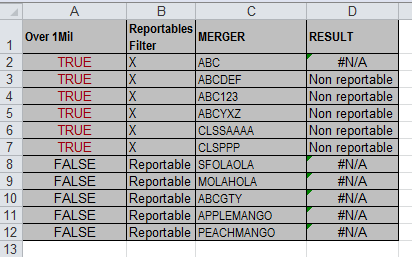# OzGrid

How to use IF and VLOOKUP formulas together

Category: [Excel]  Demo Available

### How to use IF and VLOOKUP formulas together

Requirement:

The user is requiring the following output:

3)Any N/A’s that come in the result (which would mean we need to update the Codes in sheet2)

The formula should be based on the following:

1. If col A is TRUE and Col B is X then Vlookup Col C with codes on Sheet 2 and gives the desired result AND
2. If Col A in sheet 1 is FALSE and Col B is reportable then give me reportableSolution:

=IF(AND(A2=TRUE,B2="X"),VLOOKUP(C2,Sheet2!A:B,2,0),IF(AND(A2=FALSE,B2="Reportable"),"Reportable",""))

Obtained from the OzGrid Help Forum.

Solution provided by AliGW.